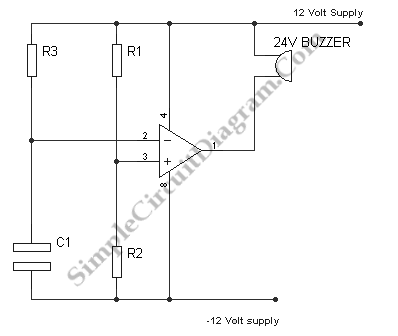# Simple Alarm Timer with Op-Amp Comparator

This timer circuit use a single op-amp which is configured as comparator, very simple, and cheap. This alarm will sound a buzzer after a period of time has elapsed since the power supply is applied. Here is the schematic diagram of the circuit:At the initial condition after the circuit is powered (you better insert a switch fro easy operation), the voltage across the capacitor is zero and the output is at +12, so the buzzer is not energized.  After a period of time, the voltage of the inverting input rises above that of the non inverting input and  the buzzer is energized (beeping) when the output goes to minus 12 volts. You can set the non inverting voltage level by choosing the values of  voltage divider R1 and R2. Finally, you can further adjust the timing by selecting an appropriate values of R3 and C1.